## Recovery Voltage:

Recovery Voltage – While closing or opening the circuit breaker, the circuit constants play an important role. It was noticed in practice a circuit breaker that would operate satisfactorily at one point on a system may not do so at another, showing that the circuit conditions have some influence on the circuit breaker behavior.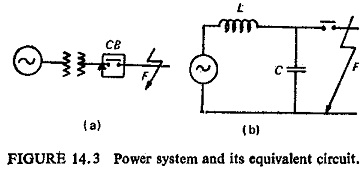Figure (14.3) shows a power system and its equivalent circuit, where L is the inductance of the generator, transformer and the transmission line and C is the capacitance of the generator, transformer, transmission line and the bushings of the circuit breaker.. The last item can be neglected at system frequency but it becomes effective at high frequencies. Overvoltages are caused by switching and also by certain faults. Figure (14.4) shows typical magnitudes of overvoltage due to switching and faults.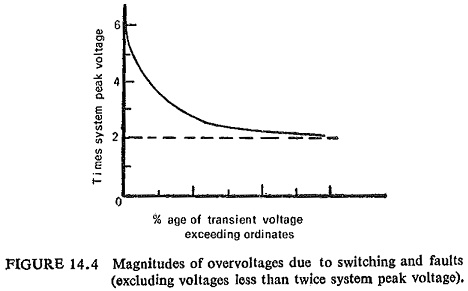The transients resulting from switching are caused by residual energy (either electric or electromagnetic or both), i.e. energy stored in capacitance C or inductance L or both, when it undergoes a change from one steady state to another owing to interruption of circuit.

### Voltage after Final Current Zero:

Experience in service and at short-circuit testing stations has demonstrated that the behaviour of circuit breakers when breaking short-circuit currents depend on a number of factors which may be termed as conditions of severity.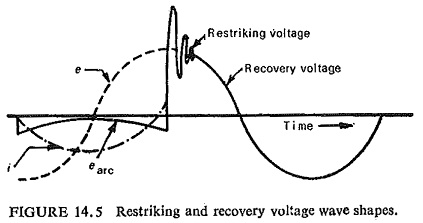The short-circuit current wave and the voltage wave (Fig. (14.5)) show that, as the current interruption takes place invariably at zero current of the current wave, the voltage that appears across the poles of the circuit breaker has an important bearing on the performance of the circuit breaker. As a matter of fact the successful interruption of the current itself is dependent on this voltage.

This voltage that appears across the poles after current interruption as seen in Fig. (14.5) has a transient part immediately after current interruption, which is known as restriking voltage and after the transient oscillations subside it attains the normal frequency voltage and then it is known as recovery voltage. These two terms are however defined as follows.

Recovery voltage: It is defined as the normal frequency rms voltage appearing between the poles of the circuit breaker after final arc extinction.

Restriking voltage: It is defined as the transient voltage that exists at or in close proximity to reach zero current pause during the arcing time.

### Conditions of Severity:

The instantaneous value of recovery voltage at the instant of final current zero depends on the following factors:

(a) Effect of Power Factor of Circuit: The instantaneous value of the recovery voltage depends on the pl. as illustrated in Fig. (14.6). It is clear therefore that a reactive short circuit is much more difficult to inter, rupt than a resistive short circuit of the same current and voltage, as the instantaneous value of the recovery voltage in the former case is high (maximum voltage) as compared to the latter (zero voltage). Unfortunately all serious short circuits are almost purely reactive.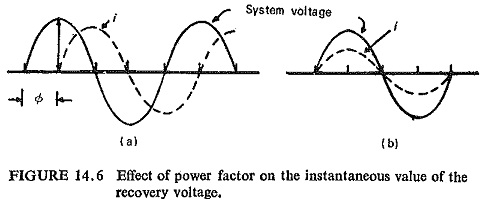(b) Effect of Circuit Conditions: The type of fault and the condition of the neutral point, i.e. whether earthed or insulated also affects the voltage across the poles of the circuit breaker in which the arc is first extinguished, This is illustrated in Fig. (14.7). If the neutral is insulated or the fault does not involve earth, a voltage of 1.5 times phase voltage appears across the pole of the circuit breaker in which the arc is first extinguished.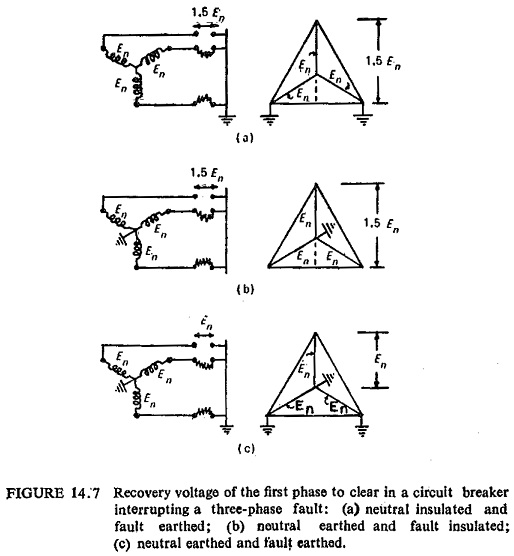(c) Current Asymmetry: With the asymmetry introduced in the current wave to be interrupted it is possible to vary the instantaneous value of the recovery voltage at current zero. This is illustrated in Fig. (14.8).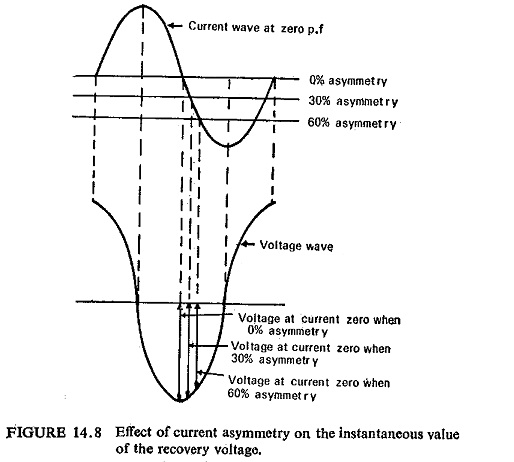The existence of a large d.c. component in a short-circuit current may cause the recovery voltage wave to start near the zero point instead of at its crest. The instantaneous value of recovery voltage is thus considerably reduced.

(d) Effect of Armature Reaction: The recovery voltage is less than the normal system voltage because of demagnetizing effect of armature reaction. The short-circuit currents are of lagging power factor and are flowing in the generator windings. They have a demagnetizing armature reaction. Hence they reduce the terminal voltage.

Scroll to Top